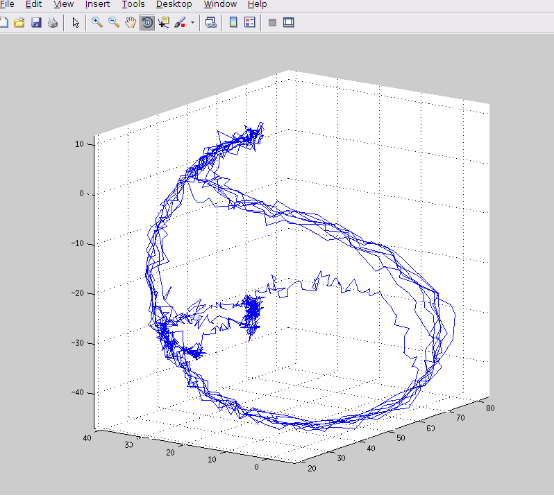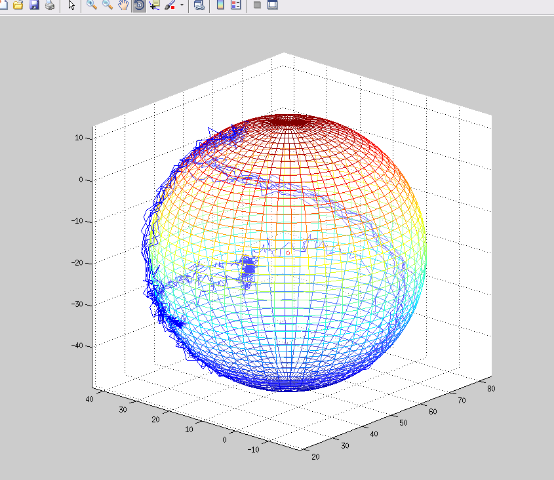# 球面拟合算法

Posted by Hu Jian on 2016-06-19

## 原理分析Pi = (Xi,Yi,Zi), i = 1，2，…，n

(x-a)^2 + (y-b)^2 + (z-c)^2 = r^2

Di^2 = (Xi-a)^2 + (Yi-b)^2 + (Zi-c)^2, i = 1，2，…，n。

## 原理扩展## 参考资料

《概率论与数理统计》（第 4 版）盛骤